# Simplified theoretical treatment of lateral structural properties under crowd excitation

## Yun Dong1, Yan An Gao2, Ye Zhu3, Peng Zhang4

1, 2, 3, 4Faculty of Architecture and Civil Engineering, Huaiyin Institute of Technology, 223001, China

1, 2, 3, 4Jiangsu Urban and Rural Residential Structure Assembly Technical Engineering Laboratory, 223001, China

1, 2Corresponding authors

Mathematical Models in Engineering, Vol. 4, Issue 4, 2018, p. 191-200. https://doi.org/10.21595/mme.2018.20177
Received 27 August 2018; accepted 6 September 2018; published 31 December 2018

Copyright © 2018 Yun Dong, et al. This is an open access article distributed under the Creative Commons Attribution License, which permits unrestricted use, distribution, and reproduction in any medium, provided the original work is properly cited.
Editor's Pick
Views 75
Abstract.

Walking crowd-induced lateral vibrations on slender structures have attracted considerable attention. The improper vibration of a structure adversely affects human comfort. To explore the effects of crowds on lateral structural properties, a pedestrian from a crowd is simulated by a walking bipedal robot. A simplified theory for structural vibration is proposed based on the assumptions of uniform distribution and synchronized walking of pedestrians. This theory can be used to describe the effect of the change in crowd size on lateral structural damping and the frequency of the structure. The method can estimate the variations in structural properties in the case of a certain crowd for engineering design. The results show that the increase in crowd size results in decrease in structural natural frequency in the lateral direction, but increases structural damping. The influence of the crowd on structural properties agrees well with the non-simplified model. Change in walking frequency has little influence on the structural properties. However, the continuous increase in crowd size on structure top causes a non-convergent amplification of dynamic response under a resonant walking excitation. This research provides a quantitative assessment on the effect of crowd size on the change in structural properties for structural design or serviceability evaluation.

Keywords: lateral vibration, dynamic properties, crowd excitation.

#### 1. Introduction

Structures tend to exhibit low frequency and damping owing to the extensive application of high-strength construction materials and slender designs. The lateral vibrational problems of flexible structures have attracted considerable attention  from several researchers since the infamous London Millennium Bridge accident . Early studies focused on vibrational measurements [3, 4] and lateral excitation force . Typical human excitation is modeled by harmonic force , which has been applied to the dynamic study of crowd-structure interaction . However, this excitation force model does not consider the dynamic contributions from humans, such as body mass or damping. A few structural measurements [8-10] have shown that humans can change dynamic structural properties such as damping or natural frequency. In addition, biomechanical measurement  has shown that the human body possesses a relatively larger damping capacity and elastic stiffness. Hence, numerous biomechanically inspired models have been presented for studying human-structure interaction dynamics. For instance, the inverted pendulum model [12, 13] has been proposed to study pedestrian–structure interaction. However, it neglects the elastic and damping capacities of pedestrians, and the corresponding gaits are unstable . Further investigations performed using equivalent reduced-DOF systems have shown that the dynamic properties of occupied structures are different from those of empty structures . Qin et al.  introduced a bipedal model with spring-damping legs for studying human–structure interaction dynamics. Gao et al. [17-19] proposed an approximate relationship between crowd size and dynamic vertical structural properties based on the bipedal model. However, no quantitative investigation has been performed on crowd size and dynamic lateral structural properties, including the contribution of dynamic crowd properties.

In order to investigate the effect of crowd size on lateral structural properties, a simplified theory model is proposed. Based on previous research , this study quantitatively explores the variation mechanism of lateral structural properties under a locomotive crowd. The crowd is simulated by walking bipedal pedestrians; it includes the contributions of mass, damping, and the stiffness property. A dynamic equation is established based on Lagrangian theory. A further simplified formula is deduced based on the assumption of uniform distribution and synchronous walking of the crowd. The formula can be quantitatively used to estimate the effect of crowd size on structural properties. This work can explain the influence of a crowd on structural properties, and it can be used to evaluate the vibrational serviceability of structures for engineering design.

#### 2.1. Dynamic equation

The pedestrian and structure are modeled using a walking bipedal model and a simple Euler-Bernoulli beam with a uniform cross section and span length ${L}_{B}$, respectively, as illustrated in Fig. 1. Figs. 1(a) and (b) show the sagittal plane coordinates ($x$-0-$z$) and frontal plane coordinates ($y$-0-$z$), respectively. The $q$th pedestrian is defined to have lump mass ${m}^{\left(q\right)}$ and walk along the right direction. ${x}^{\left(q\right)}$ and ${z}^{\left(q\right)}$ are the horizontal and vertical positions of the center of mass (CoM), respectively. ${x}_{t}^{\left(q\right)}$ and ${x}_{l}^{\left(q\right)}$ denote the longitudinal positions of the trailing and leading feet, respectively. The horizontal lateral distance between the coordinate origin and CoM is ${y}^{\left(q\right)}$, as shown in Fig. 1(b). ${L}_{\tau }^{\left(q\right)}$ represents the lateral step width of the pedestrian. ${y}_{l}^{\left(q\right)}$ and ${y}_{t}^{\left(q\right)}$ denote the lateral distance positions of the leading and trailing feet, respectively. The lateral vibrational displacement of the beam is represented by $w$.

Fig. 1. Diagram of pedestrian–structure system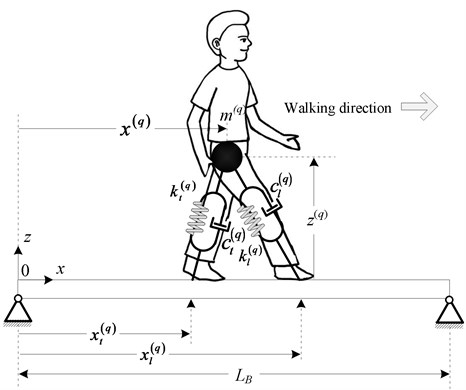a) Sagittal plane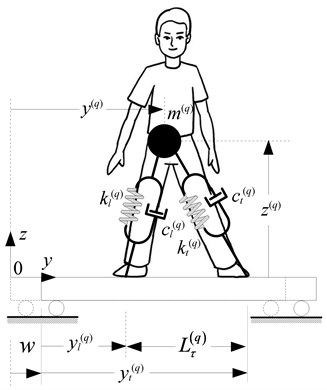b) Frontal plane

The pedestrian is assumed to walk with self-determined velocity ${\stackrel{˙}{x}}^{\left(q\right)}$  and remain in contact with the structural surface at all times. The structure is modeled by a simple supported beam with a uniform cross section (Fig. 1). The lateral dynamic equation of the pedestrian-structure system is derived based on the Lagrangian equation. The kinetic energy ($T$) and potential energy ($V$) of the system in the phase of both feet touching ground can be obtained as:

(1)
$T=\sum _{q=1}^{\chi }\sum _{\alpha ={\stackrel{˙}{x}}^{\left(q\right)},{\stackrel{˙}{y}}^{\left(q\right)},{\stackrel{˙}{z}}^{\left(q\right)}}\frac{1}{2}{m}^{\left(q\right)}{\alpha }^{2}+\frac{1}{2}{\int }_{0}^{{L}_{B}}\stackrel{-}{m}{\stackrel{˙}{w}}^{2}\left(x,t\right)dx,$
(2)
$V=\sum _{q=1}^{\chi }\sum _{\eta =l,t}\frac{1}{2}{k}_{\eta }^{\left(q\right)}{\left({L}_{0}^{\left(q\right)}-{L}_{\eta }^{\left(q\right)}\right)}^{2}+\sum _{q=1}^{\chi }{m}^{\left(q\right)}g{z}^{\left(q\right)}+\frac{1}{2}{\int }_{0}^{{L}_{B}}EI{\left(w\text{'}\text{'}\left(x,t\right)\right)}^{2}dx,$

where $\alpha$ denotes the velocity variables of CoM such as ${\stackrel{˙}{y}}^{\left(q\right)}$ in lateral vibration. ${L}_{\eta }^{\left(q\right)}$ ($\eta =l$, $t$) denotes the physical length of the leg with natural length ${L}_{0}^{\left(q\right)}$. ${k}_{\eta }^{\left(q\right)}$($\eta =l,t$) denotes the stiffness variable of the spring leg. Subscripts $l$ and $t$ indicate the leading and trailing legs, respectively. $\chi$ is the number of pedestrians or crowd size. $g$ is the gravitational acceleration. The structure is defined to have lateral bending stiffness $EI$ and longitudinal linear density$\stackrel{-}{m}\text{.}$$\stackrel{˙}{w}\left(x,t\right)=\partial w\left(x,t\right)/\partial t$ and $w\mathrm{\text{'}}\mathrm{\text{'}}\left(x,t\right)=\partial {w}^{2}\left(x,t\right)/\partial {x}^{2}$ represent the lateral vibrational velocity and curvature of the beam, respectively.

The lateral displacement of the structure can be expressed as Eq. (3) by the separation of variables:

(3)
$w\left(x,t\right)=\sum _{i=1}^{n}{\varphi }_{i}\left(x\right){Y}_{i}\left(t\right),$

where ${\varphi }_{i}\left(x\right)$ is the vibration mode that satisfies boundary conditions; ${Y}_{i}\left(t\right)$ is a generalized modal coordinate, and $n$ is the mode number of the structure considered in the analysis.

Considering the damping forces from the beam and both legs, the crowd-structure system would perform virtual work, and the variation in the virtual work, $\delta W$ is given by:

(4)
$\delta W=-\sum _{q=1}^{\chi }\sum _{\eta =l,t}{c}_{\eta }^{\left(q\right)}{\stackrel{˙}{L}}_{\eta }^{\left(q\right)}\delta {L}_{\eta }^{\left(q\right)}-{\int }_{0}^{{L}_{B}}{c}_{s}\stackrel{˙}{w\mathrm{\text{'}}\mathrm{\text{'}}}\delta {w}^{\mathrm{\text{'}}\mathrm{\text{'}}}\mathrm{d}x=\sum _{q=1}^{\chi }{Q}_{z}^{\left(q\right)}\delta {z}^{\left(q\right)}+\sum _{i=1}^{n}{Q}_{i}\delta {Y}_{i},$

where ${c}_{\eta }^{\left(q\right)}$ and ${c}_{s}$ denote leg and structural damping, respectively; ${Q}_{z}^{\left(q\right)}$ and ${Q}_{i}$ are the generalized forces of the pedestrian and structure, respectively; $\delta$ is a variational sysmbol.

The Lagrange equations of the system are given by:

(5)
$\frac{d}{dt}\left(\frac{\partial T}{\partial {\stackrel{˙}{Y}}_{i}}\right)+\frac{\partial V}{\partial {Y}_{i}}={Q}_{i}.$

Substituting Eqs. (1), (2), and (4) into Eq. (5), the equations of motion of the crowd-structure system are obtained as:

(6)

where symbol $\otimes$denotes the tensor sum of the stiffness or damping variables of the leading and trailing legs. ${\xi }_{i}$ and ${\omega }_{i}$ are the $i$th damping ratio and circular frequency of the structure in the lateral direction, respectively. The variables in Eq. (6) are given by:

(7)

where leg damping parameter ${c}_{\eta \alpha \beta }^{\left(q\right)}$ can be obtained as ${c}_{\eta \alpha \beta }^{\left(q\right)}={c}_{\eta }^{\left(q\right)}{{L}_{\eta \alpha }^{\left(q\right)}{L}_{\eta \beta }^{\left(q\right)}/\left({L}_{\eta }^{\left(q\right)}\right)}^{2}$. ${M}_{i}$ means modal mass in $i$th modal shape of structure. ${c}_{\eta \alpha \beta }^{\left(q\right)}$ denotes the damping coefficient from $\eta$ leg induced by $\alpha$ and $\beta$. ${k}_{\mathrm{\Delta }\eta }^{\left(q\right)}$ represents the stiffness of the leg $\eta$.

#### 2.2. Assumption of crowd behavior

It is noted that Eq. (6) is too complicated to describe the effect of the crowd on structural properties. To obtain a practical equation, the crowd is assumed to move with uniform walking behavior and to be evenly distributed on the structural plate (Fig. 2). The number of pedestrians in the longitudinal and lateral directions is ${N}_{x}$ and ${N}_{y}$, respectively. The distances between adjacent pedestrians in the longitudinal and lateral directions are assumed to be identical. We can obtain the distance between adjacent pedestrians in the longitudinal direction as ${L}_{B}/{N}_{x}+1$.

Fig. 2. Assumed distribution of crowd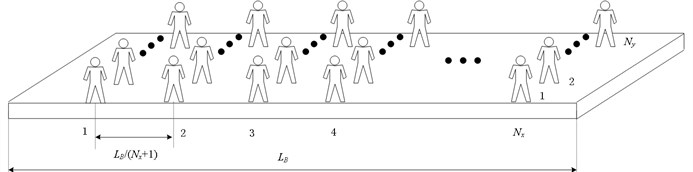Only the first modal of the structure is considered in this assumption. Step length ${L}_{\tau }^{\left(q\right)}$ is considerably less than ${L}_{B}$, that is, ${L}_{\tau }^{\left(q\right)}\ll {L}_{B}$. We can approximately define the equation ${\varphi }_{1}\left({x}_{l}^{\left(q\right)}\right)\approx {\varphi }_{1}\left({x}^{\left(q\right)}\right)\text{,}$${\varphi }_{1}\left({x}_{t}^{\left(q\right)}\right)\approx {\varphi }_{1}\left({x}^{\left(q\right)}\right)$. ${c}_{yy}^{\left(q\right)}\otimes {\mathrm{\Phi }}_{i,j}$ can be estimated using Eq. (8) considering the abovementioned approximation:

(8)

where ${L}_{ly}^{\left(q\right)}$ and ${L}_{ty}^{\left(q\right)}$ are the lateral projections of leading and trailing legs, respectively. The CoM of a pedestrian is assumed to vary as a cosine function in the lateral direction, according to the study of Liu and Urbann . The variation is assumed to follow Eq. (9):

(9)

where ${\omega }_{s}^{\left(q\right)}=\pi /{T}_{s}^{\left(q\right)}$ is the structural circular frequency, ${T}_{s}^{\left(q\right)}$ is the stepping period, and ${t}_{0}^{\left(q\right)}$ is the starting time of a new step.

After substituting Eq. (9) into ${L}_{\eta y}^{\left(q\right)}={y}^{\left(q\right)}-{y}_{\eta }^{\left(q\right)}-w\left({x}_{\eta }^{\left(q\right)},t\right)$, $\left(\eta =l,t\right)$ , we obtain:

(10)
(11)

where ${A}_{y}^{\left(q\right)}$ is assumed to the lateral swing amplitude of CoM.

Leg length is approximately equal to relax length, that is, ${L}_{l}^{\left(q\right)}\approx {L}_{0}^{\left(q\right)}$, ${L}_{t}^{\left(q\right)}\approx {L}_{0}^{\left(q\right)}$ because leg compression is considerably less than leg length. Considering that the lateral displacement of the structure is smaller than the oscillation of the CoM of a pedestrian, the lateral displacement of the structure is omitted. Eqs. (10) and (11) can be rewritten as:

(12)
(13)

Substituting Eqs. (9), (12), and (13) into Eq. (8) based on the approximations ${c}_{l}^{\left(q\right)}\approx {c}_{leg}^{\left(q\right)}$, ${c}_{t}^{\left(q\right)}\approx {c}_{leg}^{\left(q\right)}$, we can obtain:

(14)
$\sum _{q=1}^{\chi }\left({c}_{yy}^{\left(q\right)}\otimes {\mathrm{\Phi }}_{1,1}\right)={N}_{y}{c}_{leg}\left[\frac{1}{2}{\left(\frac{{L}_{w}}{{L}_{0}}\right)}^{2}+2{\left(\frac{{A}_{y}}{{L}_{0}}\mathrm{c}\mathrm{o}\mathrm{s}\left({t}_{s}{\omega }_{s}\right)\right)}^{2}\right]\sum _{q=1}^{{N}_{x}}\mathrm{s}\mathrm{i}{\mathrm{n}}^{2}\left(\frac{q\pi }{{N}_{x}+1}\right).$

The formula $\sum _{q=1}^{{N}_{x}}\mathrm{s}\mathrm{i}{\mathrm{n}}^{2}\left(\frac{q\pi }{{N}_{x}+1}\right)$ can be approximately equated to Eq. (15) using Taylor expansion:

(15)

Substituting Eq. (15) into Eq. (14), we can obtain:

(16)
$\sum _{q=1}^{\chi }\left({c}_{yy}^{\left(q\right)}\otimes {\mathrm{\Phi }}_{1,1}\right)={c}_{leg}\left[\frac{1}{2}{\left(\frac{{L}_{w}}{{L}_{0}}\right)}^{2}+2{\left(\frac{{A}_{y}}{{L}_{0}}\mathrm{c}\mathrm{o}\mathrm{s}\left({t}_{s}{\omega }_{s}\right)\right)}^{2}\right]\left(\frac{1}{3}-\frac{{\pi }^{2}}{15}+\frac{{\pi }^{4}}{252}\right){\pi }^{2}\chi .$

$\mathrm{\Delta }L$ is defined as the compression of the leg, and it is approximately given by Eq. (17):

(17)

where $\mathrm{\Delta }{z}_{g}$ is the vertical displacement of the CoM due to the gravitational effect, $z\left(t\right)$ is the vertical vibration function of the CoM, and ${A}_{z}$ is the vertical vibrational amplitude of the CoM.

Substituting Eqs. (15) and (17) into $\sum _{q=1}^{\chi }\left({k}_{\mathrm{\Delta }}^{\left(q\right)}\otimes {\mathrm{\Phi }}_{1,1}\right)$, we can obtain:

(18)

Leg damping is approximately defined as . ${c}_{xy}^{\left(q\right)}\otimes \varphi$ is approximately equal to:

(19)
${c}_{xy}^{\left(q\right)}\otimes {\varphi }_{1}\approx \mathrm{s}\mathrm{i}\mathrm{g}\mathrm{n}\left({y}_{l}^{\left(q\right)}-{y}_{t}^{\left(q\right)}\right)\frac{{L}_{s}^{\left(q\right)}{L}_{w}^{\left(q\right)}}{2{\left({L}_{0}^{\left(q\right)}\right)}^{2}}{c}_{leg}^{\left(q\right)}{\varphi }_{1}\left({x}^{\left(q\right)}\right),$

where $\mathrm{s}\mathrm{i}\mathrm{g}\mathrm{n}\left({y}_{l}^{\left(q\right)}-{y}_{t}^{\left(q\right)}\right)$ is a sign function, and it is expressed is:

(20)

Applying a similar approximation, ${c}_{yy}^{\left(q\right)}\otimes \varphi$ and ${c}_{yz}^{\left(q\right)}\otimes {\varphi }_{1}$ can be rewritten as:

(21)
(22)
${c}_{yz}^{\left(q\right)}\otimes {\varphi }_{1}\approx \mathrm{s}\mathrm{i}\mathrm{g}\mathrm{n}\left({y}_{t}^{\left(q\right)}-{y}_{l}^{\left(q\right)}\right)\frac{2{A}_{y}^{\left(q\right)}{z}^{\left(q\right)}{c}_{leg}^{\left(q\right)}}{{\left({L}_{0}^{\left(q\right)}\right)}^{2}}\mathrm{c}\mathrm{o}\mathrm{s}\left({\omega }_{s}^{\left(q\right)}{t}_{s}^{\left(q\right)}\right){\varphi }_{1}\left({x}^{\left(q\right)}\right),$
(23)

Substituting Eqs. (16), (18), (19), (21), (22), and (23) into Eq. (6), the integration of the modal function can be approximated as $\sum _{q=1}^{{N}_{x}}{\varphi }_{1}\left({x}^{\left(q\right)}\right)\approx \left(1-\frac{{\pi }^{2}}{12}\right)\frac{\pi }{2}{N}_{x}$ using Taylor expansion. We can obtain the dynamic equation of the crowd–structure system as:

(24)
${\stackrel{¨}{Y}}_{1}+2\stackrel{~}{\xi }\stackrel{~}{\omega }{\stackrel{˙}{Y}}_{1}+{\stackrel{~}{\omega }}^{2}{Y}_{1}={\stackrel{~}{F}}_{e},$

where $\stackrel{~}{\xi }$ and $\stackrel{~}{\omega }=\text{2}\pi \stackrel{~}{f}$ are the damping ratio and circular frequency of the crowd-structure system, and they are given by Eqs. (25) and (26); ${\stackrel{~}{F}}_{e}$ is the excitation acting on the crowd-structure system, and it is given by Eq. (27):

(25)
$\stackrel{~}{\xi }={\xi }_{1}\frac{{f}_{1}}{\stackrel{~}{f}}+\frac{\pi \chi {c}_{leg}}{4{M}_{1}\stackrel{~}{f}}\left[\frac{1}{2}{\left(\frac{{L}_{\tau }}{{L}_{0}}\right)}^{2}+2{\left(\frac{{A}_{y}}{{L}_{0}}\mathrm{c}\mathrm{o}\mathrm{s}\left({\omega }_{s}{t}_{s}\right)\right)}^{2}\right]\left(\frac{1}{3}-\frac{{\pi }^{2}}{15}+\frac{{\pi }^{4}}{252}\right),$
(26)
$\stackrel{~}{f}=\sqrt{{f}_{1}^{2}-\frac{\chi {k}_{\mathrm{l}\mathrm{e}\mathrm{g}}}{2{L}_{0}{M}_{1}}\left(\mathrm{\Delta }{z}_{g}+{A}_{z}\mathrm{s}\mathrm{i}\mathrm{n}\pi {f}_{s}t\right)\left(\frac{1}{3}-\frac{{\pi }^{2}}{15}+\frac{{\pi }^{4}}{252}\right)},$
(27)
${\stackrel{~}{F}}_{e}=\chi \frac{\pi }{2}\left(1-\frac{{\pi }^{2}}{12}\right)\left\{\begin{array}{l}\frac{{c}_{leg}}{2{M}_{1}{\left({L}_{0}\right)}^{2}}\left[\begin{array}{l}\mathrm{s}\mathrm{i}\mathrm{g}\mathrm{n}\left({y}_{l}-{y}_{t}\right)\left({L}_{s}{L}_{\tau }\stackrel{˙}{x}-4{A}_{y}z\stackrel{˙}{z}\mathrm{c}\mathrm{o}\mathrm{s}\left({\omega }_{s}{t}_{s}\right)\right)\\ \bullet \left({\left({L}_{\tau }\right)}^{2}+4{\left({A}_{y}\right)}^{2}\mathrm{c}\mathrm{o}{\mathrm{s}}^{2}\left({\omega }_{s}{t}_{s}\right)\right)\stackrel{˙}{y}\end{array}\right]\\ +2\mathrm{s}\mathrm{i}\mathrm{g}\mathrm{n}\left({y}_{l}-{y}_{t}\right)\frac{{k}_{leg}}{{M}_{1}}\left(\mathrm{\Delta }{z}_{g}+{A}_{z}\mathrm{s}\mathrm{i}\mathrm{n}\frac{\pi t}{{T}_{s}}\right)\frac{{A}_{y}}{{L}_{0}}\mathrm{c}\mathrm{o}\mathrm{s}\left({\omega }_{s}{t}_{s}\right)\end{array}\right\}.$

It is noted that structural damping under a crowd is related to frequency and crowd size. The damping under crowd excitation given by Eq. (25) shows that structural damping increases with damping ratio ${f}_{1}$/$\stackrel{~}{f}$ and crowd size $\chi$. However, the variation in structural damping is not linearly dependent on crowd size owing to the complicated relationship between crowd size and structural frequency (Eq. (26)). The frequency of the structure under crowd excitation (Eq. (26)) indicates that the variation in the structure is related to crowd size and walking frequency. A larger crowd size would result in smaller frequency. It is more important that this simplified theory can quantitatively describe the effect of a crowd on structural properties. The effect of a crowd on the dynamic structural characteristics under a certain crowd size can be precisely estimated. This theory can provide practical guidance for the servicing assessment and design of slender structures in future.

#### 3. Numerical verification

To verify the proposed model, a footbridge with a span length of 100 m (Carroll et al., ) is used to calculate the effect of the crowd on structural properties. The lateral dam-ping and fundamental frequency of the footbridge are $\xi =$ 0.5 % and ${f}_{1}=$ 0.85 Hz, respectively. The ratio of the masses of the crowd and structure is linearly changed from ${M}_{p}$/${M}_{s}=$ 0.0 to 0.5. Different stepping frequencies are selected for simulation. The calculation duration is 1 min. Figs. 3(a) and (b) show the lateral displacement and acceleration responses under resonance excitation, respectively. Figs. 3(c) and (d) show the lateral displacement and acceleration responses under${f}_{s}=\text{2}{f}_{1}$, respectively. Compared to excitation under${f}_{s}=\text{2}{f}_{1}$, resonance excitation can trigger increasing responses.

Fig. 3. Effect of ratio of masses of crowd and structure on the structural responses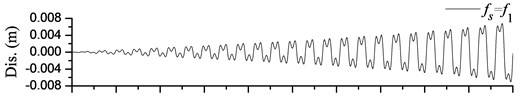a)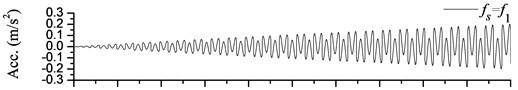b)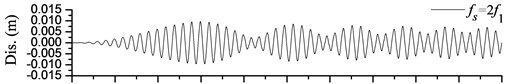c)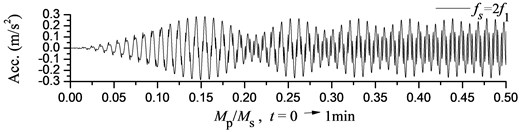d)

To investigate the effect of crowd excitation on structural properties, the peak responses and dynamic structural characteristics under different stepping frequencies are compared as shown in Fig. 4. Fig. 4(a) shows the peak accelerations under variable mass ratio ${M}_{p}$/${M}_{s}$. Comparison to non-resonant frequency ${f}_{s}=\text{2}{f}_{1}$, the acceleration of the structure increases with mass ratio. A larger crowd size is more unfavorable under resonant excitation. However, the change in excitation has negligible effects on lateral structural properties. Structural frequency decreases as mass ratio increases (Fig. 4(b)).

Fig. 4. Effect of ratio of masses of crowd and structure on structural properties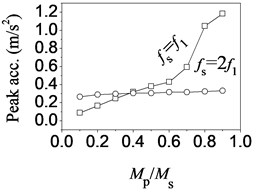a)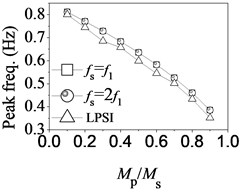b)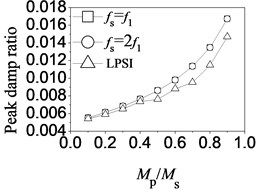c)

However, it does not change under different excitation frequencies. The structural damping ratio increases with mass ratio (Fig. 4(c)). However, it does not change under different excitation frequencies. Thus, it can be seen that the change in excitation affects only structural response and has no influence on dynamic structural properties. In addition, comparisons to the original non-simplified model  (LPSI) show that the peak frequency (Fig. 4(b)) and damping ratio (Fig. 4(c)) of the simplified model are closely with the non-simplified model (LPSI). The influence characteristics of the crowd on the structural lateral properties agree with measurement , that crowd can improve the lateral damping capacity, but deteriorate lateral frequency of structure.

To further explore the effect of larger crowd size under resonant excitation, the ratio between the masses of the crowd and structure is increased from zero to the maximum (1). The structural frequency under varying mass ratio is plotted in Fig. 5(a), and it decreases as mass ratio increases. A larger mass ratio results in lower frequency, which implies that a larger crowd size leads to increase in lateral structural unstable risk. On the contrary, a larger mass ratio results in a higher damping ratio (Fig. 5(b)). Thus, it can be seen that the crowd evidently affects lateral structural properties. A larger crowd size leads to lower frequency; however, this is favorable for damping capacity. The lateral acceleration of the midspan is shown in Fig. 5(c). The increase in mass ratio leads to increase in response under crowd resonant excitation. This implies that resonant excitation and increasing crowd size result in the amplification of structure responses.

Fig. 5. Dynamic properties under crowd resonant frequency ${f}_{s}=\text{2}{f}_{1}$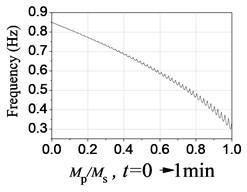a)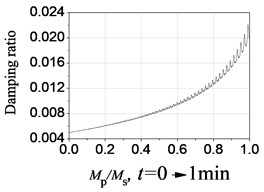b)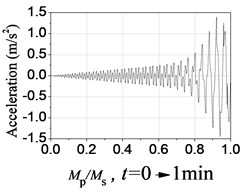c)

The authors declare that there is no conflict of interest regarding the publication of this paper.

#### 4. Conclusions

This paper proposes a new theory for predicting lateral structural properties under bipedal crowd excitation. Even though the simplified theory is based on the assumption of uniform distribution of pedestrians with consistent walking behavior, it can quantitatively describe the variations in lateral structural properties in theory. The lateral frequency and damping ratio of the structure can be obtained for a given crowd size. As crowd size increases, the lateral frequency of the structure decreases and the damping of the structure increases. The change in walking frequency has little influence on the structural properties. Resonant excitation and increasing crowd size result in the amplification of structural responses. The proposed theory for walking pedestrians opens the field for further exploration of the effect of walking crowds on the vibrational and dynamic properties of slender structures. It provides a quantitative assessment on the effect of crowd size on the change in structural properties for structural design or serviceability evaluation.

#### Acknowledgements

This research is supported by the Major Projects of Natural Science Research in Jiangsu Colleges and Universities (17KJA560001), National Natural Science Foundation of China (51808247), Natural Science Foundation of University in Jiangsu Province (18KJB560003), the Natural Science Foundation of Jiangsu Province (BK20160426), the Science and Technology planning project of Jiangsu Province (BY2016061-29), the Natural Science Foundation for Colleges and Universities in Jiangsu Province (17KJB130003) and the Project with Science and Technology of Huai’an City (HAG201606). The help from Ding Zhou is also acknowledged.

1. Ingolfsson E. T., Georgakis C. T., Jönsson J. Pedestrian-induced lateral vibrations of footbridges: A literature review. Engineering Structures, Vol. 45, 2012, p. 21-52. [Publisher]
2. Newland D. E. Vibration of the London Millennium Bridge: cause and cure. International Journal of Acoustics and Vibration, Vol. 8, 2003, p. 9-14. [Publisher]
3. Nakamura S. I., Kawasaki T. Lateral vibration of footbridges by synchronous walking. Journal of Constructional Steel Research, Vol. 62, 2006, p. 1148-1160. [Publisher]
4. Brownjohn J. M. W., Pavic A. Experimental methods for estimating modal mass in footbridges using human-induced dynamic excitation. Engineering Structures, Vol. 29, 2007, p. 2833-2843. [Publisher]
5. Ricciardelli F., Pizzimenti A. D. Lateral walking-induced forces on footbridges. Journal of Bridge Engineering, Vol. 12, 2007, p. 677-688. [Publisher]
6. Zivanovic S., Pavic A., Reynolds P. Vibration serviceability of footbridges under human-induced excitation: a literature review. Journal of Sound and Vibration, Vol. 279, 2005, p. 1-74. [Publisher]
7. Carroll S. P., Owen J. S., Hussein M. F. M. Modeling crowd-bridge dynamic interaction with a discretely defined crowd. Journal of Sound and Vibration, Vol. 331, 2012, p. 2685-2709. [Publisher]
8. Brownjohn J. M. W. Energy dissipation in one-way slabs with human participation. Proceedings of the Asia-Pacific Vibration Conference, Nanyang Technological University, Singapore, Vol. 1, 1999, p. 155-160. [Search CrossRef]
9. Ellis B. R., Ji T., Littler J. D. The response of grandstands to dynamic crowd loads. Proceedings of the Institution of Civil Engineers – Structures and Buildings, Vol. 140, 2000, p. 355-365. [Publisher]
10. Shahabpoor E., Pavic A., Racic V., Zivanovic S. Effect of group walking traffic on dynamic properties of pedestrian structures. Journal of Sound and Vibration, Vol. 387, 2017, p. 207-225. [Publisher]
11. Hong H., Kim S., Kim C., Lee S., Park S. Spring-like gait mechanics observed during walking in both young and older adults. Journal of Biomechanics, Vol. 46, 2013, p. 77-82. [Publisher]
12. Bocian M., Macdonald J. H. G., Burn J. F. Biomechanically inspired modeling of pedestrian-induced forces on laterally oscillating structures. Journal of Sound and Vibration, Vol. 311, 2012, p. 3914-3929. [Publisher]
13. Carroll S. P., Owen J. S., Hussein M. F. M. A coupled biomechanical/discrete element crowd model of crowd-bridge dynamic interaction and application to the Clifton Suspension Bridge. Engineering Structures, Vol. 49, 2013, p. 58-75. [Publisher]
14. Macdonald J. H. G. Lateral excitation of bridges by balancing pedestrians. Proceedings of The Royal Society A, Vol. 465, 2009, p. 1055-1073. [Publisher]
15. Sim J., Blakeborough A., Williams M. Modeling of joint crowd-structure system using equivalent reduced-DOF system. Shock and Vibration, Vol. 14, 2007, p. 261-270. [Publisher]
16. Qin J. W., Law S. S., Yang Q. S., Yang N. Pedestrian-bridge dynamic interaction, including human participation. Journal of Sound and Vibration, Vol. 332, 2013, p. 1107-1124. [Publisher]
17. Gao Y. A., Yang Q. S., Qin J. W. Bipedal crowd-structure interaction including social force effect. International Journal of Structural Stability and Dynamics, Vol. 17, 2017, p. 1750079. [Publisher]
18. Gao Y. A., Wang J., Liu M. The vertical dynamic properties of flexible footbridges under bipedal crowd induced excitation. Applied Sciences, Vol. 7, 2017, p. 1-10. [Publisher]
19. Gao Y. A., Yang Q. S. A theoretical treatment of crowd-structure interaction. International Journal of Structural Stability and Dynamics, Vol. 18, Issue 1, 2018, p. 187001. [Publisher]
20. Yang Q. S., Gao Y. A. A theory treatment of pedestrian-induced lateral vibration of structure. Journal of Dynamic Systems, Measurement, and Control, Vol. 140, 2018, p. 61004. [Publisher]
21. Liu J., Urbann O. Bipedal walking with dynamic balance that involves three-dimensional upper body motion. Robotics and Autonomous Systems, Vol. 77, 2016, p. 39-54. [Publisher]
22. Brownjohn J. M. W., Fok P., Roche M., Moyo P. Long span steel pedestrian bridge at Singapore Changi Airport – part 1: prediction of vibration serviceability problems. The Structural Engineer, Vol. 82, 2004, p. 21-27. [Search CrossRef]

#### Cited By

 Mathematical Models in Engineering Yan An Gao, Wei-Dong Zhang, Abdoullah Namdar, Ye Zhu 2019